## Bubble Sort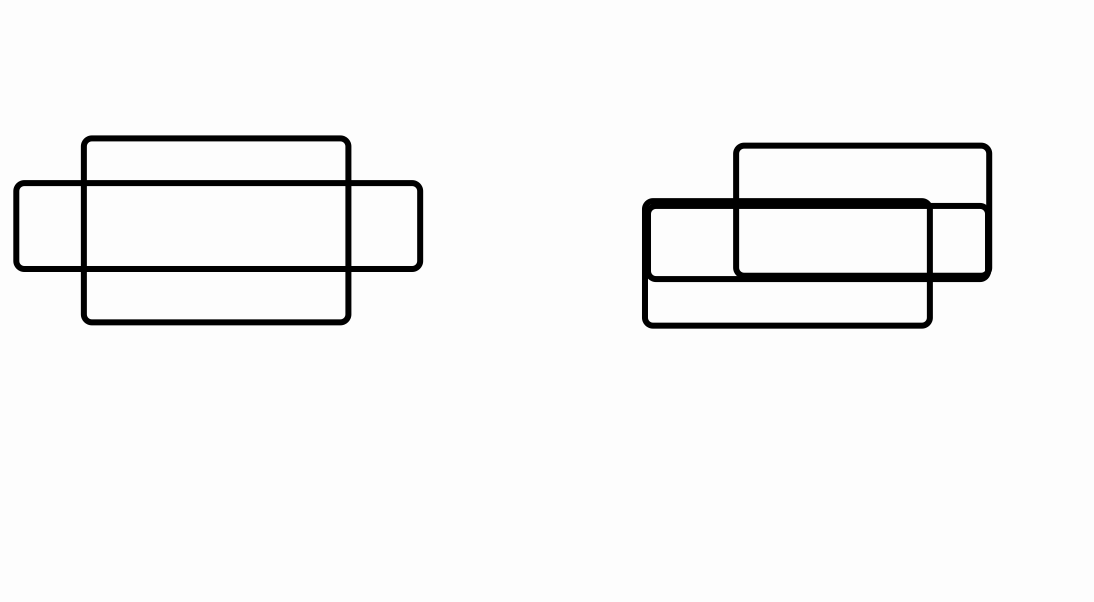## P5902 [IOI2009]salesman## BZOJ3340 [CEOI2013] Tram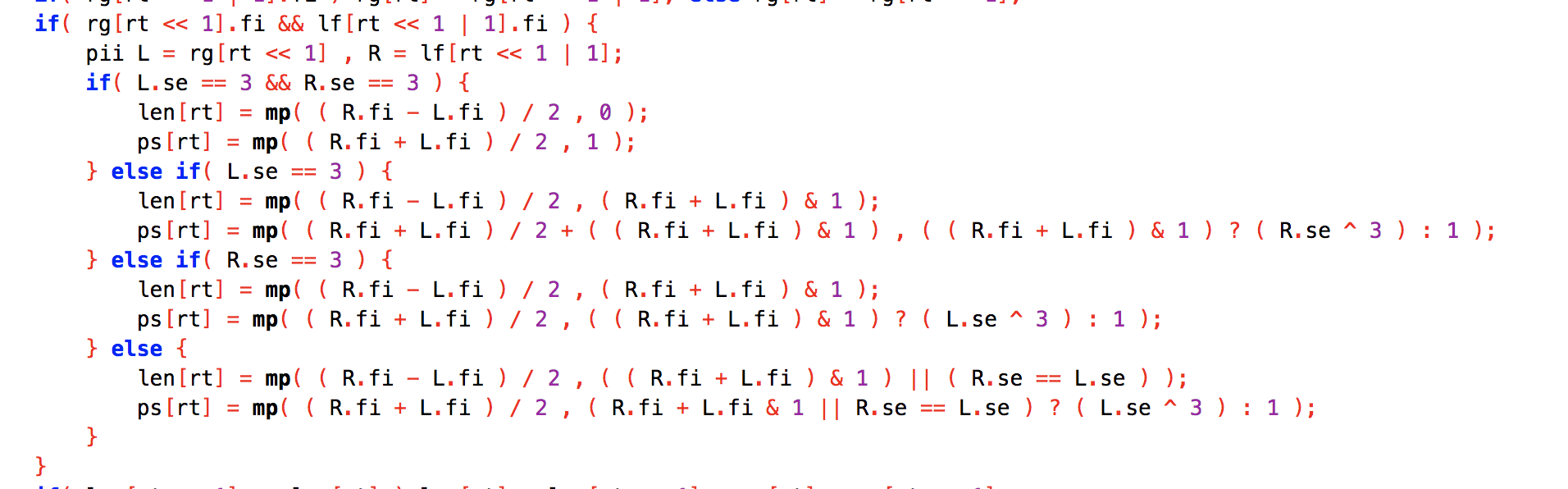## T1 calxor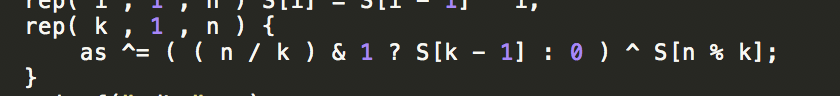## T4 editor

• 插入，我们维护出当前光标的权值，然后直接插入即可，插入后继续维护光标位置。
• 删除，直接在 splay 删除。
• 左右移，移动光标位置即可。
• 询问，把 $[l,r]$ 放到一个子树上，然后维护个子树和即可。
• 修改，把这个数找到并且 splay 到根修改即可。

## Circle Union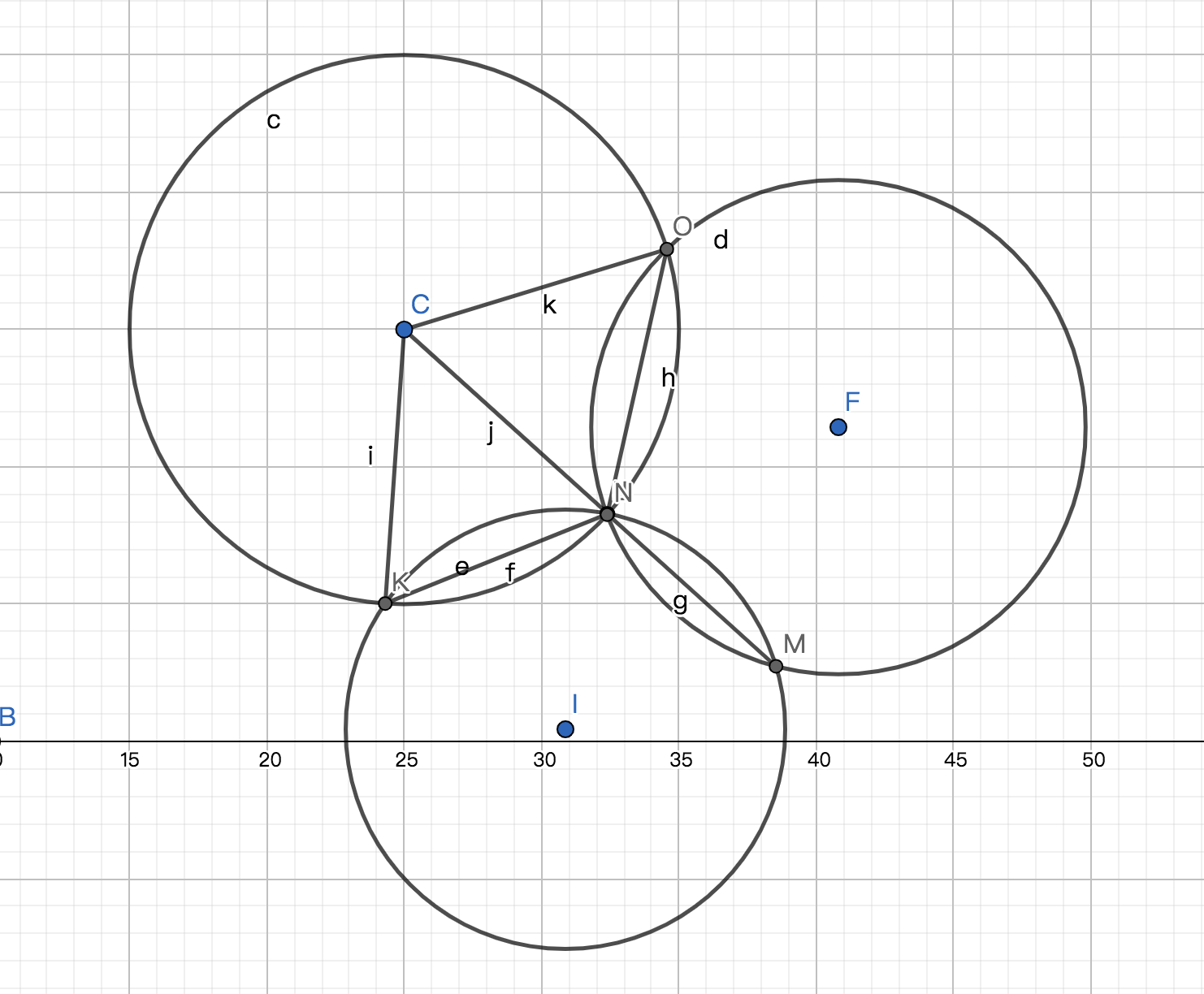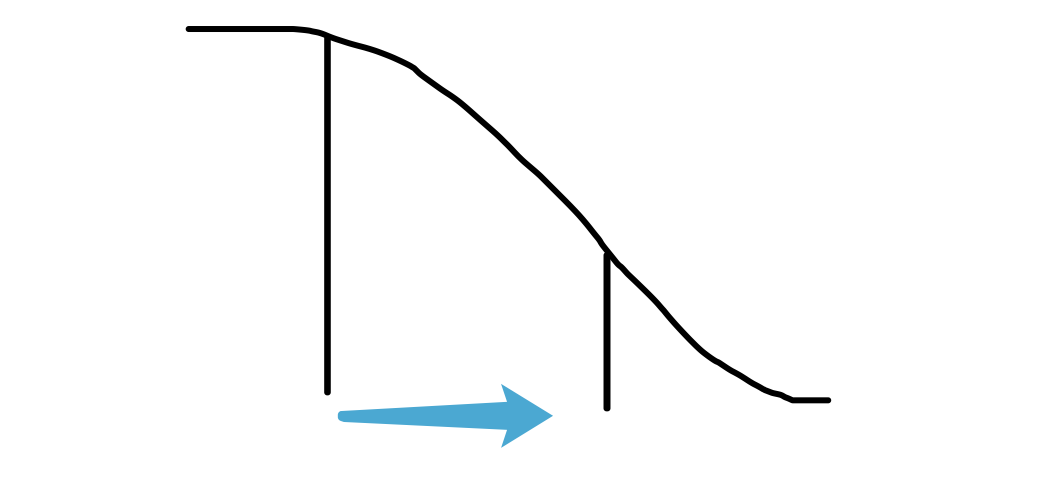## CF1425E

• 用了两个激发

那么一定可以通过一次操作构造出权值的和减去最便宜的两个开关。

构造方法就是，假装最便宜的两个开关是 $i,j$ 且满足 $i < j$ ，那么我们从 $j-1$ 向 $1$ 连边形成环，于是就可以通过 $D_i + D_j$ 获得所有的 $A_i$

• 只用一个激发

• 抛弃 $A_n$

不难发现最优决策一定是在前面找最便宜的开关，获得 $\sum_{i < n} A_i$ 。因为我们不可能通过 $D_n$ 来达成抛弃 $A_n$ ，所以这样花费和收益都最优化了。

• 不抛弃 $A_n$

只能连一次，所以肯定是选择一个段后缀，但是不一定可以拿到这段后缀。

• 如果最后我们不是选择 $D_1$ ，那么一定可以拿完 $\sum_{i \ge k} A_i$ ，因为可以直接把 $E_1$ 连向 $3$ 。

• 如果我们最后选择的是 $D_1$ ，那么必然是抛弃中间最小的 $A_k$ 。

## ARC045D

• 如果这个点连出两个非树边，不影响联通性。
• 如果这个点只连出了一个非树边，直接删掉这个边和他的父亲边，显然不会影响联通性。
• 如果这个点没连出非树边，那么考虑它父亲的另一个儿子，这个儿子显然不会连出去非树边，删掉这两个边即可。
• 如果这个点没连出非树边，并且他的父亲没有其他儿子，那么直接删掉这个点，这个点的父亲，以及这个点的父亲到父亲的父亲的边。因为随时边数都是偶数，所以显然找得到。